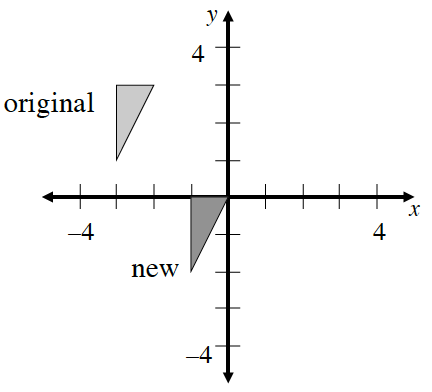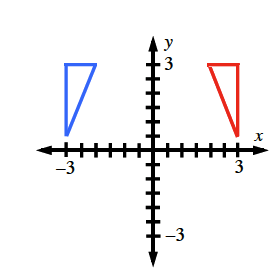### Home > CC2MN > Chapter 6 Unit 6A > Lesson CC3: 6.1.4 > Problem6-36

6-36.Sketch the graph below on your paper.

1. Write directions to translate the original triangle to make the new triangle.

Examine the translation of the triangle as a horizontal and vertical part. Did the triangle shift left or right? Up or down?

Use arrows drawn on the graph as indicators for the direction of the translation.

1. What are the coordinates of the vertices (corners) of the new shape?

The coordinate of a given point is denoted $(x,y)$:
The distance on the $x$-axis and the distance on the $y$-axis from the origin.

Try using the horizontal ($x$-axis) and vertical ($y$-axis) arrows for help in writing the coordinates. Remember that movement left or down is negative (toward a 'negative' direction).

$(−1,0),(0,0),(−1,−2)$

2. On your graph, reflect the original triangle across the $y$‑axis. What are the coordinates of the new triangle?

What changes about the coordinate points when a shape is reflected across the $y$-axis?

The original triangle is in blue, the reflected triangle in red.
Write the coordinates for each vertex of the new (red) triangle.